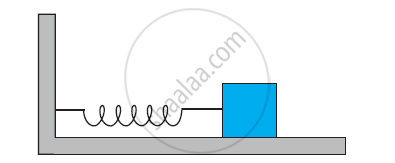CBSE (Science) Class 11CBSE
Share

# A Spring Having with a Spring Constant 1200 N M–1 Is Mounted on a Horizontal Table as Shown in Fig. a Mass of 3 Kg is Attached to the Free End of the Spring. the Mass is Then Pulled Sideways to a Distance of 2.0 Cm and Released. Determine (I) the Frequency of Oscillations, (Ii) Maximum Acceleration of the Mass, and (Iii) the Maximum Speed of the Mass. - CBSE (Science) Class 11 - Physics

ConceptSome Systems Executing Simple Harmonic Motion

#### Question

A spring having with a spring constant 1200 N m–1 is mounted on a horizontal table as shown in Fig. A mass of 3 kg is attached to the free end of the spring. The mass is then pulled sideways to a distance of 2.0 cm and released.Determine (i) the frequency of oscillations, (ii) maximum acceleration of the mass, and (iii) the maximum speed of the mass.

#### Solution 1

Spring constant, k = 1200 N m–1

Mass, = 3 kg

Displacement, A = 2.0 cm = 0.02 cm

(i) Frequency of oscillation v, is given by the relation:

v = 1/T = 1/(2pi) sqrt(k/m)

Where, T is the time period

:. v = 1/(2xx3.14) sqrt(1200/3) = 3.18 "m/s"

Hence, the frequency of oscillations is 3.18 cycles per second.

ii) Maximum acceleration (a) is given by the relation:

a = ω2 A

Where

ω = Angular frequency  = sqrt(k/m)

A = Maximum displacement

:. a = k/m A = (1200xx0.02)/(3) =  8 ms^(-2)

Hence, the maximum acceleration of the mass is 8.0 m/s2

iii) Maximum velocity, vmax = Aω

= A sqrt(k/m) = 0.02 xx sqrt(1200/3) = 0.4 "m/s"

Hence, the maximum velocity of the mass is 0.4 m/s.

#### Solution 2

K = 1200 Mn^(-1); m = 3.0 kg, a= 2.0 cm = 0.02 m

i) Frequency, v =  1/T = 1/(2pi) sqrt(k/m) = 1/(2xx3.14) sqrt(1200/3) = 3.2 s^(-1)

ii) Acceleration, A = omega^2 " " y = k/m  y

Acceleration will be maximum when y is maximum i.e y = q

:. max acceleration, A_"max" = (ka)/m =(1200xx0.02)/3 = 8 ms^(-2)

iii) Max speed of the mass will be when it is passing throught mean position

V_"max" = aomega = sqrt(k/m) = 0.02 xx sqrt(1200/3) = 0.4 ms^(-1)

Is there an error in this question or solution?

#### Video TutorialsVIEW ALL 

Solution A Spring Having with a Spring Constant 1200 N M–1 Is Mounted on a Horizontal Table as Shown in Fig. a Mass of 3 Kg is Attached to the Free End of the Spring. the Mass is Then Pulled Sideways to a Distance of 2.0 Cm and Released. Determine (I) the Frequency of Oscillations, (Ii) Maximum Acceleration of the Mass, and (Iii) the Maximum Speed of the Mass. Concept: Some Systems Executing Simple Harmonic Motion.
S